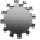Conference TopicsContributions related, but not limited, to the following topics of interest are welcome:

Four colour theorem
History of Topology
Non-Euclidean geometry
Topology and Scottish mathematical physics
Cubic surfaces
Mathematics and art - perspective
Euclid's definitions
Fractal Geometry
Absolute geometry
Affine geometry
Algebraic geometry
Analytic geometry
Archimedes' use of infinitesimals
Birational geometry
Complex geometry
Combinatorial geometry
Computational geometry
Conformal geometry
Constructive solid geometry
Contact geometry
Convex geometry
Descriptive geometry
Differential geometry
Digital geometry
Discrete geometry
Distance geometry
Elliptic geometry
Enumerative geometry
Epipolar geometry
Euclidean geometry
Finite geometry
Geometry of numbers
Hyperbolic geometry
Information geometry
Integral geometry
Inversive geometry
Inversive ring geometry
Klein geometry
Lie sphere geometry
Non-Euclidean geometry
Numerical geometry
Ordered geometry
Parabolic geometry
Plane geometry
Projective geometry
Quantum geometry
Riemannian geometry
Ruppeiner geometry
Spherical geometry
Symplectic geometry
Synthetic geometry
Systolic geometry
Taxicab geometry
Toric geometry
Transformation geometry
Tropical geometry
Topology and physics
Topology and dynamical systems